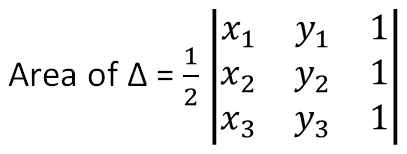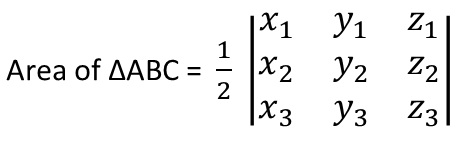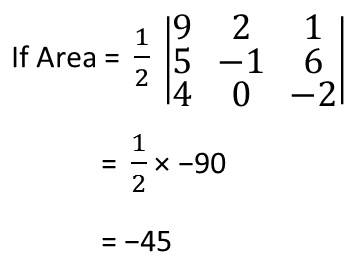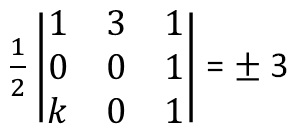In a 2D plane,

For three points

A (x 1 , y 1 ),

B (x 2 , y 2 ),

C (x 3 , y 3 )## Find area of triangle with vertices (3, 8), (-4, 2), (5, 1)

Check solution - Example 17

## For points in 3D plane

A (x 1 , y 1 , z 1 )

B (x 2 , y 2 , z 2 )

C (x 3 , y 3 , z 3 )There are some points to note:-

1. If Area of triangle = 0,
then the three points are collinear

2. If value of determinant comes negative, we will take the
positive value as area

ExampleTherefore,
Area = 45 square units

3. .If area is given,
We take both positive and negative value of determinant
Example
If Area = 3 square unitsSubscribe to our Youtube Channel - https://you.tube/teachoo

1. Chapter 4 Class 12 Determinants
2. Concept wise
3. Area of triangle

Transcript

Area of ∆ = 1/2 |■8(𝑥_1&𝑦_1&1@𝑥_2&𝑦_2&1@𝑥_3&𝑦_3&1)| For points in 3D plane A (x1, y1, z1) B (x2, y2 , z2) C (x3, y3 , z3) Area of ∆ABC = 1/2 |■8(𝑥_1&𝑦_1&𝑧_1@𝑥_2&𝑦_2&𝑧_2@𝑥_3&𝑦_3&𝑧_3 )| Example If Area = 1/2 |■8(9&2&1@5&−1&6@4&0&−2)| = 1/2 × −90 = −45 Therefore, Area = 45 square units 1/2 |■8(1&3&1@0&0&1@𝑘&0&1)| = ± 3

Area of triangle